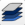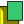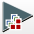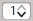# 6.2. Tutorial: Image Conversion to Reflectance¶

This tutorial is about the image conversion to Radiance at the Sensor’s Aperture or to Top Of Atmosphere (TOA) Reflectance. It is assumed that one has the basic knowledge of SCP and Basic Tutorials.

SCP includes several tool for preprocessing images such as Landsat, Sentinel-2, Sentinel-3. Using satellite images from various sources could require the preprocessing and radiometric correction.

Usually, remote sensing images are delivered as calibrated Digital Numbers (DN), and the conversion to radiance or reflectance can be performed through parameters that are provided with the image.

This tutorials aims to describe how to perform the conversion to TOA reflectance of remote sensing images. The calculation can be performed for all the bands at once in Band calc, knowing the required parameters.

Following the video of this tutorial.

## 6.2.1. Input Data¶

In this tutorial, we are going to use a subset of a Landsat Satellites image (data available from the U.S. Geological Survey). You can download the sample image from this archive (about 4 MB) that includes bands 2, 3, 4, 5, 6, and 7.

We’ll pretend that Landsat tool is not included in the preprocessing tools of SCP, but use the Band calc to perform the calculations for all the bands at once. The same tutorial could be applied to different satellite images, knowing the equations and factors required for the image conversion to reflectance.

Start QGIS and the SCP.

Open the tab Band set clicking the buttonin the SCP menu or the SCP dock. Click the buttonand open the directory containing the input bands and select all the .tif files. The selected bands will be added to the active band set.

In the table Band set definition order the band names in ascending order (clickto sort bands by name automatically). Finally, select Landsat 8 OLI from the list Wavelength quick settings, in order to set automatically the Center wavelength of each band and the Wavelength unit (required for spectral signature calculation). If the satellite image wasn’t listed in Wavelength quick settings it is possible to enter manually the Center wavelength.

## 6.2.2. Conversion to reflectance¶

Usually, image providers describe the equations required for converting the DN to reflectance (or radiance) values. For Landsat 8 images TOA planetary reflectance ($$\rho_{\lambda}$$) is given by (https://www.usgs.gov/core-science-systems/nli/landsat/using-usgs-landsat-level-1-data-product):

$\rho_{\lambda} = M_{p} * Q_{cal} + A_{p}$

where:

• $$M_{p}$$ = Band-specific multiplicative rescaling factor from Landsat metadata (REFLECTANCE_MULT_BAND_x, where x is the band number)
• $$A_{p}$$ = Band-specific additive rescaling factor from Landsat metadata (REFLECTANCE_ADD_BAND_x, where x is the band number)
• $$Q_{cal}$$ = Quantized and calibrated standard product pixel values (DN)

A similar equation is available for the conversion to Radiance at the Sensor’s Aperture.

TIP : the conversion performed by SCP also includes a correction for solar angle, please read Top Of Atmosphere (TOA) Reflectance.

Now, we need to open the metadata file LC08_L1TP_015033_20170416_20170501_01_T1_MTL.txt (the matadata file name could vary for other images) using a text editor and take note of the REFLECTANCE_MULT_BAND_x and REFLECTANCE_ADD_BAND_x values for each band (bands 2, 3, 4, 5, 6, 7). In this case the values are:

Landsat Bands REFLECTANCE_MULT_BAND REFLECTANCE_ADD_BAND Band number in Band Set
Band 2 0.00002 -0.1 1
Band 3 0.00002 -0.1 2
Band 4 0.00002 -0.1 3
Band 5 0.00002 -0.1 4
Band 6 0.00002 -0.1 5
Band 7 0.00002 -0.1 6

Therefore, we can write the expressions to be used in the Band calc. We can write the expressions using the Input variables referred to the band number in active Band set (i.e. bandset#b BAND_NUMBER), thus we can use the same expressions later for other images of the same satellite. For instance, the following variable refers to band 1 of the active Band set:

"bandset#b1"


Therefore, the equation $$\rho_{\lambda} = M_{p} * Q_{cal} + A_{p}$$ becomes the following expression for the first band:

"bandset#b1" * 0.00002 - 0.1


We also use the Output variables in order to set automatically the output names of each calculation. The variable #BANDSET# allows for using the name (without the ending number) of the first band in the Band set.

Output names can be defined in the expression line entering the symbol @ followed by the name, so the previous expression for band 1 becomes:

"bandset#b1" * 0.00002 - 0.1 @CON_#BANDSET#2


In the above expression, the output name will have the prefix CON_ followed by the original image name and the original band number (i.e. 2).

We can write the expressions for all the bands (in this case it is simple because the multiplicative and additive rescaling factors are the same for each band):

"bandset#b1" * 0.00002 - 0.1 @CON_#BANDSET#2
"bandset#b2" * 0.00002 - 0.1 @CON_#BANDSET#3
"bandset#b3" * 0.00002 - 0.1 @CON_#BANDSET#4
"bandset#b4" * 0.00002 - 0.1 @CON_#BANDSET#5
"bandset#b5" * 0.00002 - 0.1 @CON_#BANDSET#6
"bandset#b6" * 0.00002 - 0.1 @CON_#BANDSET#7


We can now enter all the above expressions in Band calc and click the button RUNto select a directory where bands are saved and start the calculations.

After the process, all the converted images are added to the map, as you can see with the file name CON_ + original image name + band number .

Well done! We have converted to reflectance all the bands of remote sensing image that could be used for performing a land cover classification.

## 6.2.3. Save the expressions in Band Calc (optional)¶

We can save the expressions in the list of Functions for a rapid use. This requires the creation of a text file containing the expressions. The structure of the text file must be in the form expression_name; expression (separated by ;) where the expression_name is the name that is displayed in the list Functions (for instance Landsat conversion).

Because we have multiple expressions, we must put every expression in the same line and separate by <br /> such as:

Landsat conversion; "bandset#b1" * 0.00002 - 0.1 @CON_#BANDSET#2 <br />"bandset#b2" * 0.00002 - 0.1 @CON_#BANDSET#3 <br />"bandset#b3" * 0.00002 - 0.1 @CON_#BANDSET#4 <br />"bandset#b4" * 0.00002 - 0.1 @CON_#BANDSET#5 <br />"bandset#b5" * 0.00002 - 0.1 @CON_#BANDSET#6 <br />"bandset#b6" * 0.00002 - 0.1 @CON_#BANDSET#7


First, we open a text editor and paste the above string. After saving the text file (.txt), in Band calc click the buttonand select the saved text file containing the function. The item Landsat conversion will be listed under Custom. Double clicking this item will add the expressions to the calculation.

The same expressions could be used for converting other images having the same multiplicative and additive rescaling factors. Of course, in case of different satellites we should adapt the expressions to the specific factors and equations provided with the images. We could also create more complicated expressions, for instance adding the calculation of spectral indices such as NDVI. This will be described in other tutorials.

## 6.2.4. Scale the output to reduce file size (optional)¶

The results of the previously calculated bands were saved as float values (i.e. Float32) and as you can see pixel values have several decimal places. If we would like to reduce file size, we can reduce the decimal precision by reducing the bits of data type. For instance, the precision provided by 4 decimal places could be sufficient in several cases.

We can use the scale option in Band calc to create a raster which is scaled and evaluated as float by software (the decimal places depend on the scale factor). The data type Int16 can store values from −32,768 to 32,767, therefore dividing pixel values by 10,000 we could get a range with 4 decimal places from −3.2768 to 3.2767 , which is coherent to the value range of reflectance (i.e. from 0 to 1) or other spectral indices.

In Band calc under Output raster select Int16 , checkSet scaleand enter 0.0001 . This means that original raster values will be divided by 0.0001 and saved as 16bit integer. When the raster is read by software, pixels will be multiplied on the fly by 0.0001 obtaining the value with 4 decimal places.

The rasters of data type Int16 defined with decimal scale value will be interpreted as Float32 by software. For example, a pixel value 0.596812 is first divided by 0.0001 , converted to integer, and stored as 5968. When the raster is processed by software, pixel value is multiplied on the fly by 0.0001 obtaining the value 0.5968 .

Now we can perform the same calculations described in Conversion to reflectance obtaining rasters of about half the file size of the previous ones, and of course reduced decimal precision.

Other calculations with Band calc will described in the next few tutorials.